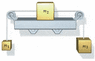# Boxes on a frictionless shelf

• makerfeldt
In summary, a box of mass m2 = 3.7 kg is attached by strings to boxes of masses m1 = 1.2 kg and m3 = 2.1 kg, with both pulleys being frictionless and massless. The system is released from rest and the relevant forces of gravity are 18.62 on m1 and 27.44 on m3. However, the information provided is incomplete as the diagram is missing and it is not specified if m1 and m3 are hanging off opposite sides of the shelf. To solve the problem, the free-body diagrams for each box should be drawn and Newton's laws should be applied.

## Homework Statement

A box of mass m2 = 3.7 kg rests on a frictionless horizontal shelf and is attached by strings to boxes of masses m1 = 1.2 kg and m3 = 2.1 kg as shown below. Both pulleys are frictionless and massless. The system is released from rest. After it is released, find the following.

## The Attempt at a Solution

I found the relevant forces of gravity to be 18.62 on m1, and 27.44 on m3, but I have little clue of where to go from there. Any idea would be greatly appreciated.

makerfeldt said:

## Homework Statement

A box of mass m2 = 3.7 kg rests on a frictionless horizontal shelf and is attached by strings to boxes of masses m1 = 1.2 kg and m3 = 2.1 kg as shown below.
missing diagram?

Both pulleys are frictionless and massless. The system is released from rest. After it is released, find the following.
incomplete information.
Are m1 and m3 hanging off opposite sides of the shelf?

## The Attempt at a Solution

I found the relevant forces of gravity to be 18.62 on m1, and 27.44 on m3, but I have little clue of where to go from there. Any idea would be greatly appreciated.
Draw a free-body diagram for each box and apply Newton's laws: ƩF=ma

My apologies; the boxes m1 and m3 are indeed hanging off opposite sides of the shelf.

#### Attachments

•4-p-067.gif
7.2 KB · Views: 419
OK - so did you draw a free body diagram for each box?